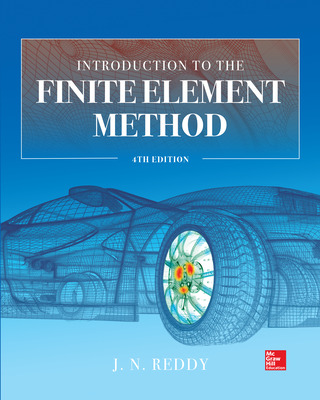# Introduction to the Finite Element Method 4E

4th Edition
By J. Reddy
ISBN10: 1259861902
ISBN13: 9781259861901

## Purchase Options

Students, we’re committed to providing you with high-value course solutions backed by great service and a team that cares about your success. See tabs below to explore options and pricing. Don't forget, we accept financial aid and scholarship funds in the form of credit or debit cards.

### Product

The estimated amount of time this product will be on the market is based on a number of factors, including faculty input to instructional design and the prior revision cycle and updates to academic research-which typically results in a revision cycle ranging from every two to four years for this product. Pricing subject to change at any time.

### Program Details

1 General Introduction
1.1 Background
1.2 Mathematical Model Development
1.3 Numerical Simulations
1.4 The Finite Element Method
1.5 The Present Study
1.6 Summary
Problems

2 Mathematical Preliminaries and Classical Variational Methods
2.1 General Introduction
2.2 Some Mathematical Concepts and Formulae
2.3 Energy and Virtual Work Principles
2.4 Integral Formulations of Differential Equations
2.5 Variational Methods
2.6 Equations of Continuum Mechanics
2.7 Summary
Problems

3 1-D Finite Element Models of Second-Order Differential Equations
3.1 Introduction
3.2 Finite Element Analysis Steps
3.3 Finite Element Models of Discrete Systems
3.4 Finite Element Models of Continuous Systems
3.5 Axisymmetric Problems
3.6 Errors in Finite Element Analysis
3.7 Summary
Problems

4 Applications to 1-D Heat Transfer and Fluid and Solid
Mechanics Problems
4.2 Heat Transfer
4.3 Fluid Mechanics
4.4 Solid and Structural Mechanics
4.5 Summary
Problems

5 Finite Element Analysis of Beams and Circular Plates
5.1 Introduction
5.2 Euler–Bernoulli Beam Element
5.3 Timoshenko Beam Elements
5.4 Axisymmetric Bending of Circular Plates
5.5 Summary
Problems

6 Plane Trusses and Frames
6.1 Introduction
6.2 Analysis of Trusses
6.3 Analysis of Plane Frame Structures
6.4 Inclusion of Constraint Conditions
6.5 Summary
Problems

7 Eigenvalue and Time-Dependent Problems in 1-D
7.1 Introduction
7.2 Equations of Motion
7.3 Eigenvalue Problems
7.4 Transient Analysis
7.5 Summary
Problems

8 Numerical Integration and Computer Implementation
8.1 Introduction
8.2 Numerical Integration
8.3 Computer Implementation
8.4 Applications of Program FEM1D
8.5 Summary
Problems

9 Single-Variable Problems in Two Dimensions
9.1 Introduction
9.2 Boundary Value Problems
9.3 Modeling Considerations
9.4 Numerical Examples
9.5 Eigenvalue and Time-Dependent Problems
9.6 Summary
Problems

10 2-D Interpolation Functions, Numerical Integration, and Computer Implementation
10.1 Introduction
10.2 2-D Element Library
10.3 Numerical Integration
10.4 Modeling Considerations
10.5 Computer Implementation and FEM2D
10.6 Summary
Problems

11 Flows of Viscous Incompressible Fluids
11.1 Introduction
11.2 Governing Equations
11.3 Velocity–Pressure Formulation
11.4 Penalty Function Formulation
11.5 Computational Aspects
11.6 Numerical Examples
11.7 Summary
Problems

12 Plane Elasticity
12.1 Introduction
12.2 Governing Equations
12.3 Virtual Work and Weak Formulations
12.4 Finite Element Model
12.5 Elimination of Shear Locking in Linear Elements
12.6 Numerical Examples
12.7 Summary
Problems